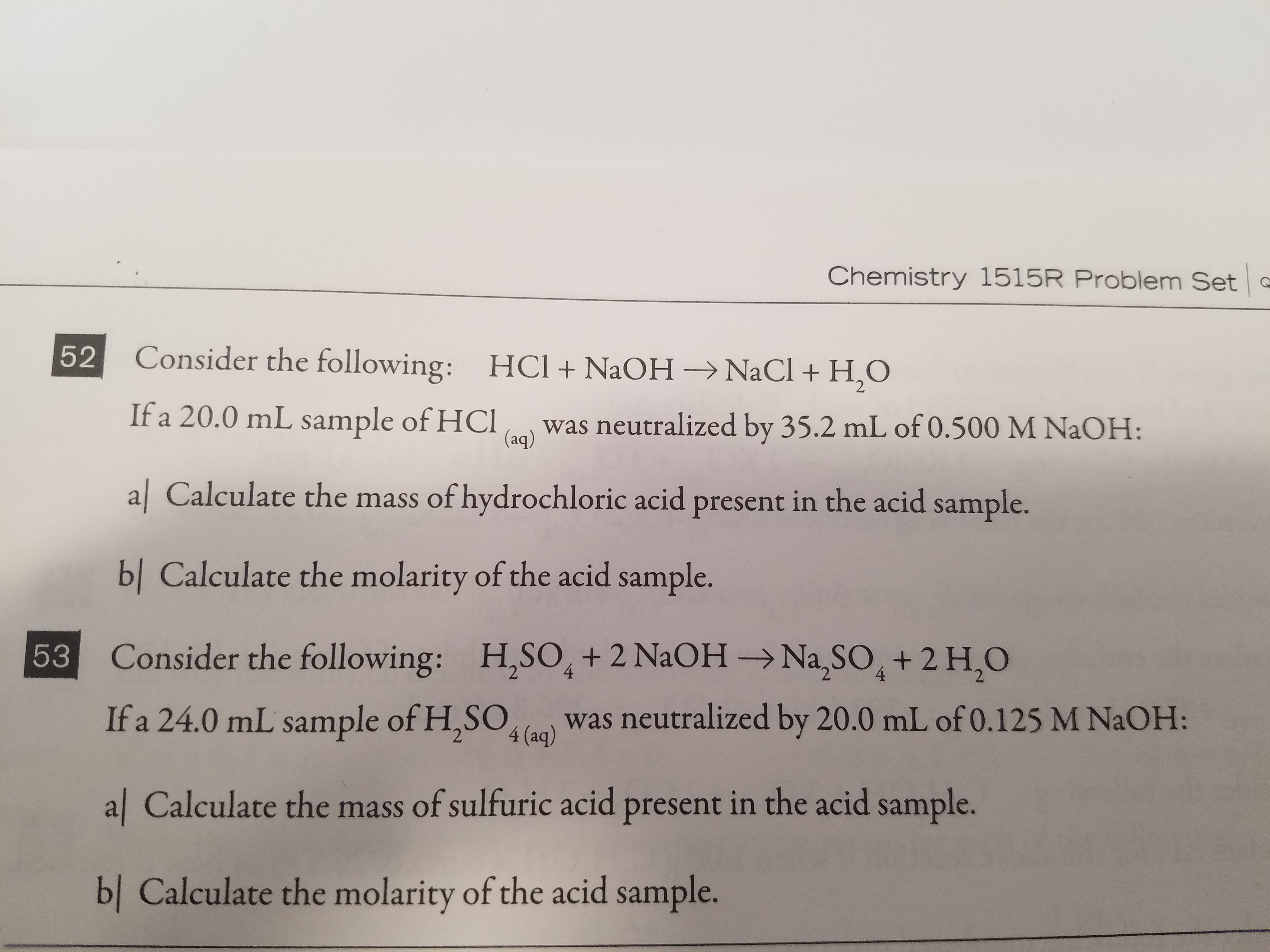# Chemistry 1515R Problem Set Consider the following: HCl + NaOH → NaCl + H2O If a 20.0 mL sap H was neutralized by 35.2 mL of 0.500 M NaOH: al Calculate the mass of hydrochloric acid present in the acid sample. bl Calculate the molarity of the acid sample. Consider the following: If a 24.0 mL sample of H.SOlo) was neutralized by 20.0 mL of 0.125 M NaOH: al Calculate the mass of sulfuric acid present in the acid sample. 52 (aq) ES H,so, + 2 NaOH → Na2SO4 + 2 H,O 53 bl Calculate the molarity of the acid sample.

Questionhelp_outlineImage TranscriptioncloseChemistry 1515R Problem Set Consider the following: HCl + NaOH → NaCl + H2O If a 20.0 mL sap H was neutralized by 35.2 mL of 0.500 M NaOH: al Calculate the mass of hydrochloric acid present in the acid sample. bl Calculate the molarity of the acid sample. Consider the following: If a 24.0 mL sample of H.SOlo) was neutralized by 20.0 mL of 0.125 M NaOH: al Calculate the mass of sulfuric acid present in the acid sample. 52 (aq) ES H,so, + 2 NaOH → Na2SO4 + 2 H,O 53 bl Calculate the molarity of the acid sample. fullscreen

### Want to see the step-by-step answer?